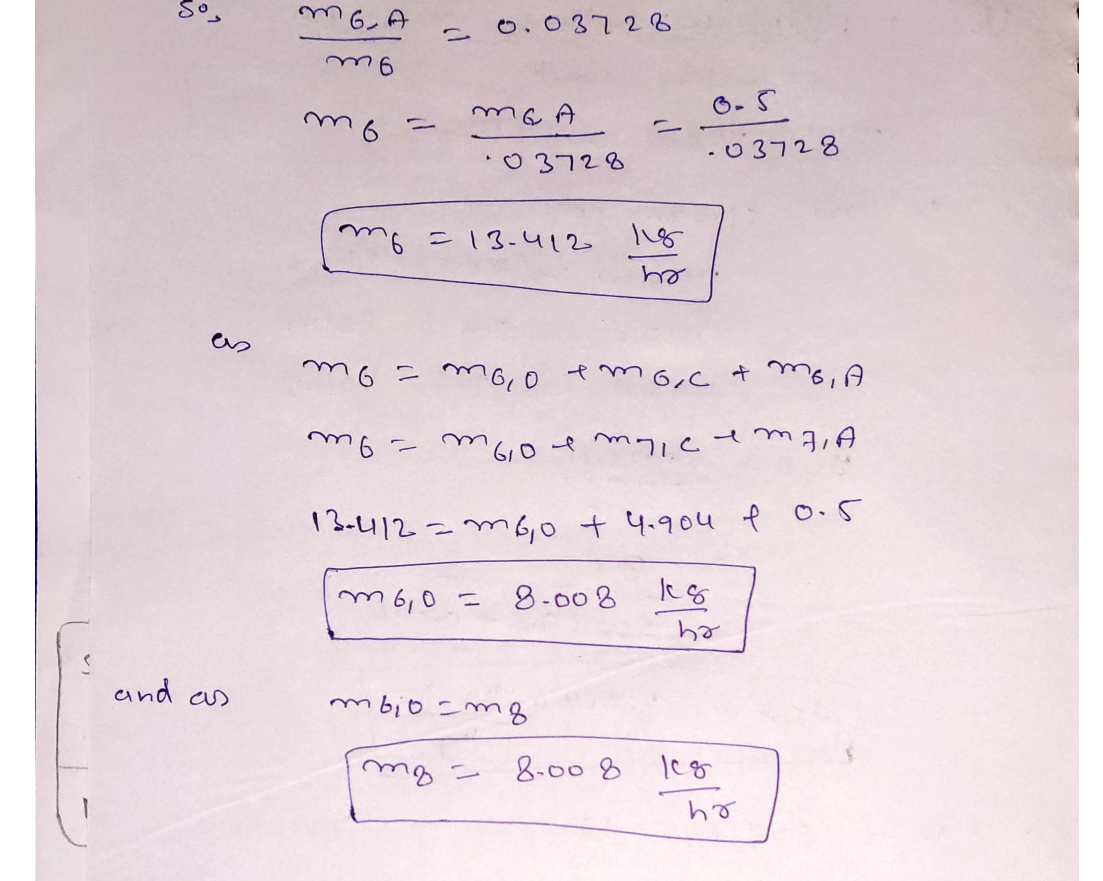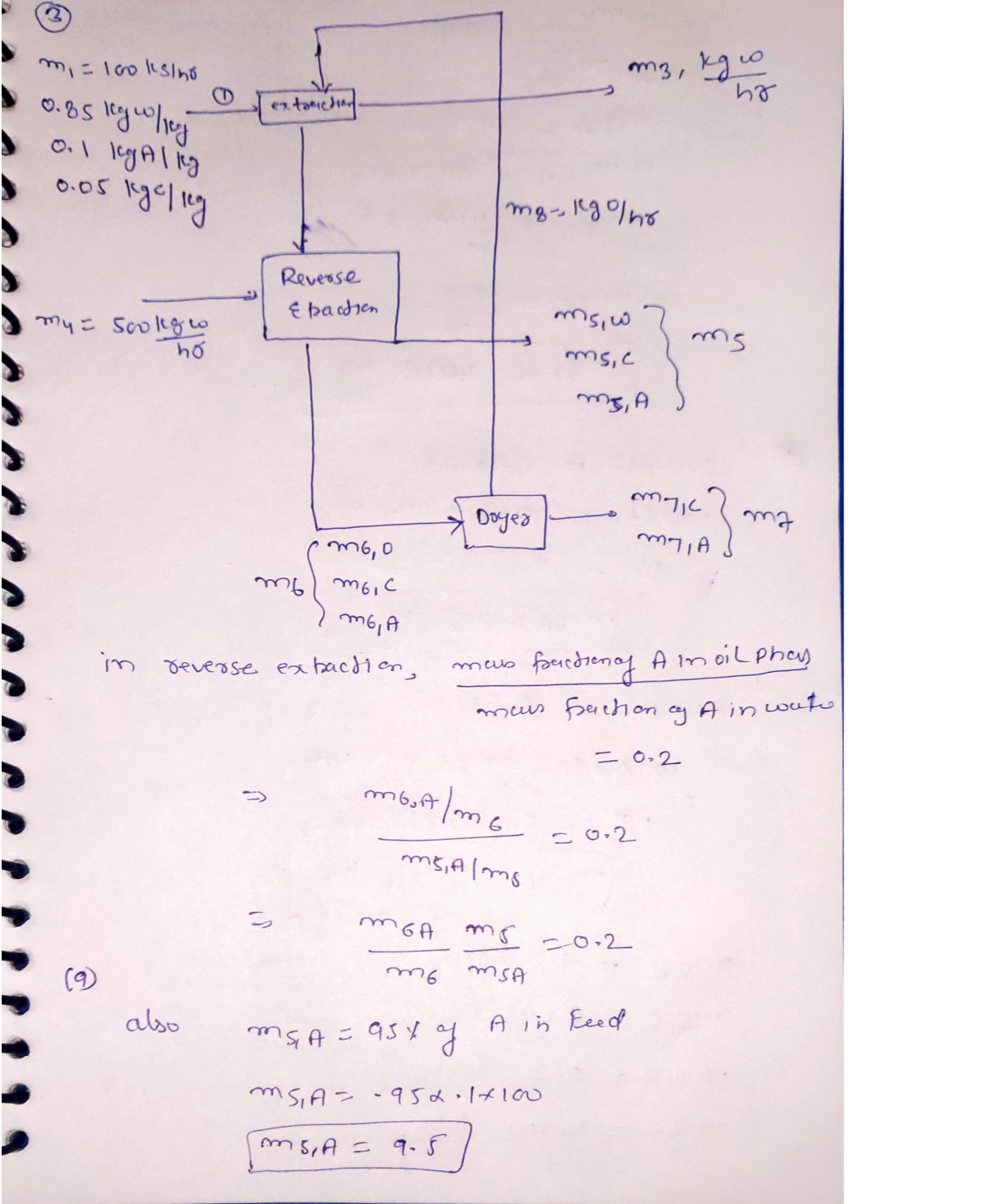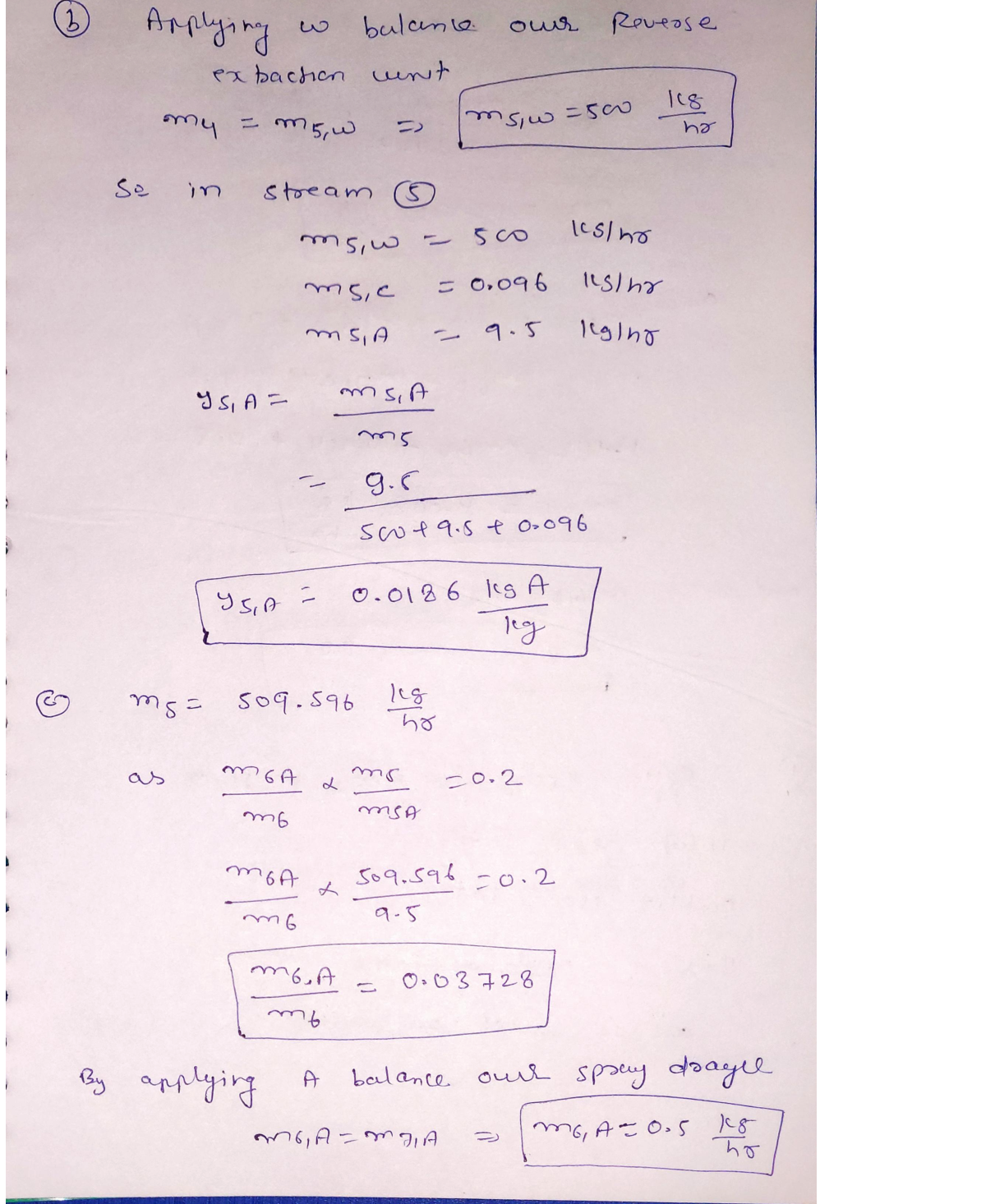Question

Mass Transfer

(50 pts; 2 pages) Material balance without reaction. Below is a simplification of a purification process for antibiotics produced by fermentation. Consider an aqueous stream containing an antibiotic (A) and a contaminant (C) (Stream 1 on below process flow diagram). The antibiotic is purified by first extracting out into an oil that is immiscible with water; the contaminant is also extracted into this oil. Then, a reverse extraction occurs where water at a higher pH selectively extracts out the deprotonated form of the antibiotic.The distribution coefficient for the antibiotic in this reverse extraction is mass fraction A im oil phase – 0.2. The aqueous stream 5 leaving the reverse extraction unit mass fraction A in water contains 95% of the antibiotic freshly fed into the overall process (stream 1), and the purity of this antibiotic relative to the contaminant is 99% on a mass basis (e.g. water is not included in this calculation).

(32 pts) Solve for the mass amounts of antibiotic and contaminant leaving the system

Determine the mass fraction of antibiotic in stream 5.

Determine the mass flow rate of oil (mg) needed to recirculate in the system.D/hr):Verified### Question 36209Mass Transfer

(60 pts; 3 pages) Energy balance with reaction. Among common liquid fuels, acetylene(C2H2) torches burn at the highest adiabatic flame temperatures. It turns out that for our specific application we desire an outlet temperature of 1500°C, which is much cooler than the adiabatic flame temperature. 1500°C is enforced by using a large quantity of excess air.Assume that acetylene burns completely and clean and with a basis of calculation of 1mol/s, determine the % of excess air by solving an energy balance. The inlet acetylene and air gas streams are at 25°C and 1 atm, and the outlet is set at 1500°C and 1 atm.
a. (4 pts) Explain conceptually why increasing air flow rate lowers the adiabatic flame temperature.
b. (8 pts) Determine the theoretical moles of air necessary to completely combust 1 mol/sof acetylene.
c. (28 pts) Determine the unknown inlet and outlet specific enthalpies (and the heat of reaction if using heat of reaction method). To help your calculations, please use the inlet-outlet enthalpy table below and explicitly state your solution method and reference state(s) directly on this sheet!!
d. (12 pts) Write the appropriate form of the energy balance needed to solve for n1 – the unknown molar flow rate of air. Your answer should have n1 on the left hand side of the equation and all remaining knowns and unknowns on the process flow diagram on the right hand side.

### Question 36208Mass Transfer

(50 pts; 2 pages) Material balance without reaction. Below is a simplification of a purification process for antibiotics produced by fermentation. Consider an aqueous stream containing an antibiotic (A) and a contaminant (C) (Stream 1 on below process flow diagram). The antibiotic is purified by first extracting out into an oil that is immiscible with water; the contaminant is also extracted into this oil. Then, a reverse extraction occurs where water at a higher pH selectively extracts out the deprotonated form of the antibiotic.The distribution coefficient for the antibiotic in this reverse extraction is mass fraction A im oil phase – 0.2. The aqueous stream 5 leaving the reverse extraction unit mass fraction A in water contains 95% of the antibiotic freshly fed into the overall process (stream 1), and the purity of this antibiotic relative to the contaminant is 99% on a mass basis (e.g. water is not included in this calculation).
(32 pts) Solve for the mass amounts of antibiotic and contaminant leaving the system
Determine the mass fraction of antibiotic in stream 5.
Determine the mass flow rate of oil (mg) needed to recirculate in the system.D/hr):

### Question 36207Mass Transfer

(50 pts; 2 pages) Material balance with reaction. Consider the production of acetylene gas(C2H2) from solid calcium carbide (CaC2) and water. 100 mol/min of a stream of 95 mol %solid calcium carbide and the balance inerts is fed to a reactor at 60°C. Acetylene gas and calcium hydroxide (Ca(OH)2) are the products of the reaction, and the single pass conversion is 100% with respect to calcium carbide. Two streams leave the reactor inequilibrium at 60°C and 1 atm - a liquid stream containing unreacted water and calcium hydroxide and inerts, and a gas stream containing acetylene and water vapor. The inerts and calcium hydroxide have negligible vapor pressure (P* close to zero). Determine the molar flow rate of water fed into the reactor by answering the following questions.
a. (16 pts) Label the process flow diagram as completely as possible. If you know the amount (or the amount is zero), label it. Otherwise, use a question mark (?) to the right of the variable to indicate that the variable is unknown.
b. (34 pts) Write the complete set of algebraic equations that can be used to solve for these unknowns listed on part a. Do not solve the equations! Remember that your only variables in the below equations should be the unknowns you listed in part a.

### Question 36206Mass Transfer

(40 pts; 2 pages) Energy balance without reaction. Researchers at Michigan State University have developed a sustainable process for converting biomass to sugars that can then be converted to fuels and chemicals. This process involves using liquid ammonia (NH3)at high temperatures and pressures to pretreat the biomass. Consider the recycling process for the spent ammonia at the pilot scale. Spent ammonia vapor at 130°C and 40 bar is condensed and compressed back to liquid at 120°C and 100 bar. The condenser removes 1.45 kW of heat from the inlet ammonia stream. Determine the work in hp required by the compressor. The heat capacity of ammonia in liquid phase is 0.0808 kJ/mol/°C and assume that heat capacity is independent with temperature. The specific gravity of liquid ammonia is0.604.
a. (20 pts) Determine the unknown specific enthalpies H1 and A2 in units of kJ/kg. To help your calculations, please use the inlet-outlet enthalpy table below and explicitly state your reference states!
(20 pts) Set up and simplify the appropriate form of the energy balance equation. Use this to solve for the work added to the system by the compressor in units of hp.

### Question 33636Mass Transfer

Hot oil (c, 2200 J/kg.K) is to be cooled by water (cp 4180 J/kg.K) in a 2-shell-passes and "N-tube-passes" heat exchanger. The tubes are thin-walled and are made of copper with a diameter of 1.8 cm. The length of each tubepass in the heat exchanger is "L" m, and the overall heat transfer coefficient is 340 W/m?.K. Water flows through the tubes at a total rate of 0.1 kg/s, and the oil through the shell at a rate of 0.2 kg/s. The water and the oil enter at temperatures 18 °C and 160 °C, respectively. Design a heat exchanger if it is desired to heat the water to 80 °C.

### Question 27775Mass Transfer

A wastewater steam is discharged from chemical processing plant and it flow through a channel of length L, depth h and width W to a water treatment facility. It is desired to estimate the rate of oxygen absorption by the liquid waste stream that flows in the channel. The initial concentration of oxygen in the wastewater stream (when it leaves the processing plant) is Co.
Express the equation in (2) in dimensionless form. List the important dimensionlessgroups and their physical meaning.
Indicate the boundary conditions needed to solve the mass transfer equation for the liquid phase.
Simplify the mass transfer equation to an equation in two-dimensional coordinates.Indicate the reasons for your choice of coordinates.
Write the appropriate mass transfer equation (for the liquid phase) assuming that the liquid velocity profile in the axial direction is uniform (i.e., plug-flow). What are the appropriate boundary conditions?
Write the complete mass transfer equation for oxygen transport in the channel.

### Question 27279Mass Transfer

The Coronavirus pandemic has witnessed an increase in the use of disinfectants, such as household bleach. This is an aqueous alkaline solution of sodium chloride (NaCl) and sodium hypochlorite (NaCIO), and is a powerful oxidising agent.
Acidic solutions of hypochlorous acid (HCIO) are made when chlorine gas(Cl2) dissolves in water. Reaction of the chlorine with water is considered to be fast.
(a)Write down the reaction between chlorine and water.
If the reaction between chlorine gas and water occurs as a single step, explain why the reaction you have written in part (a) can be considered to be overall pseudo-first order.(b)
(c)Write down the time-dependent reaction-diffusion equation relevant to this problem in extractive reaction.
D is the diffusion coefficient of chlorine in water.
(e)Using your answers to parts (c) and (d) and showing all of your steps, demonstrate that the steady-state concentration profile of chlorine in water is given by equation (5):
\frac{c}{c_{\mathfrak{\theta}}}=\exp \left(-x \sqrt{\frac{k_{-}}{D}}\right)
where:
c is the concentration of chlorine in water,
co is the concentration of chlorine in water at the water/gas interface.
x is the distance in the water from the water/gas interface,
k, is the pseudo-first order rate constant for the reaction of chlorine with water, and
(d)What is meant by the term steady-state?
In experimental studies using a wetted-wall absorption column, themass transfer coefficient for chlorine into water is 200 um s.(f)
i. Explain what is meant by the term wetted-wall absorption column, and describe how they differ from packed tower absorption columns.
ii. What advantages do wetted-wall columns have over packed towers?
iii. Given the diffusion coefficient of chlorine in water is1.25 x 10 cm? s', determine the pseudo-first order rate constant for the reaction between chlorine and water.

### Question 27278Mass Transfer

Rectangular channel flow reactors are a useful basis for the development of electro chemical synthesis as part of a green and sustainable chemical manufacturing involving process electrification.
A laboratory-scale electrochemical reactor, developed as a rectangular channel flow cell, had length (L) of 30 cm, a cross-sectional width (B) of3.0 cm and a cross-sectional height (s) of 5.0 mm, and was designed so that the flow would be well-developed before it reached the electrodes embedded in the sides of the reactor. Operation of the cell occurred isothermally at293 K, for a liquid electrolyte (of viscosity 1.002 mPa s and density998.2 kg m) for the electrochemical conversion of a reactant. The diffusion coefficient of the reactant in the liquid electrolyte is 2.67 x 10 cm? s at293 K.
(a)Explaining all terms and symbols used, define the following.
What are the units of kinematic viscosity (v)?kinematic viscosity of the liquid electrolyte at 293 K, providing your answer in the units you have previously given.(b)Calculate the
If the equivalent diameter (de) of the laboratory reactor is given byequation (1),(c)
\mathrm{d}_{\mathrm{e}}=4 \times \frac{\text { cross-sectional area }}{\text { cross-sectional perimeter }}
calculate the value of the equivalent diameter of the reactor, andgive its units.
(d)Noting that the Reynolds number (Re) of the reactor is given byequation (2),
\mathrm{Re}=\frac{\mathrm{d}_{e} \mathrm{u}}{\mathrm{v}}
in which u is the mean electrolyte velocity, use the data provided tocalculate, at 293 K, both the Reynolds number and the Schmidtnumber as a function of the volume flow rate. Comment on yourresults.
(e)Use the data provided to calculate the Sherwood number (Sh) as a function of the volume flow rate at 293 K, and then plot Ig(Sh)against Ig(Re) and comment on your plot.
For Re < 2000, the Sherwood number is predicted to be related tothe Reynolds number and the Schmidt number by equation (3)(f)
S h=c R e^{d} S c
where a and b are constants. Use your answers to parts (d) and (e)to calculate the values of a and b. Comment on your findings.
For Re > 2000, the Sherwood number is predicted to be related tothe Reynolds number and the Schmidt number by equation (4)
\mathrm{Sh}=\mathrm{aRe}^{\mathrm{b}} \mathrm{Sc}^{/ / 3}
where c and d are constants. Use your answers to parts (d) and (e)to calculate the values of c and d. Comment on your findings.

### Question 12795Mass Transfer

iii. Given the diffusion coefficient of chlorine in water is1.25 x 10° cm? s", determine the pseudo-first order rate constant for the reaction between chlorine and water.
ii. What advantages do wetted-wall columns have over packed towers?
In experimental studies using a wetted-wall absorption column, the mass transfer coefficient for chlorine into water is 200 um s.s1.
i. Explain what is meant by the term wetted-wallabsorption column, and describe how they differ frompacked tower absorption columns.

### Question 12794Mass Transfer

Using your answers to parts (c) and (d) and showing all of your steps, demonstrate that the steady-state concentration profile ofchlorine in water is given by equation (5):
\frac{c}{c_{0}}=\exp \left(-x \sqrt{\frac{k_{r}}{D}}\right)

### Submit query